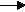gcsescience.com                                                 21                                                 gcsescience.com

Atomic Structure

State Symbols used in Chemical Equations.

The equations from the two previous pages
(3)    2
Cl22KCl

(4)   4Li  +  O22Li2O
still require state symbols to be complete.

The complete equations in words and symbols would be
potassium + chlorinepotassium chloride.
2K(s)  +    Cl2(g)2KCl(s)

lithium + oxygenlithium oxide.
4Li(s)  +    O2(g)2Li
2O(s)

The state symbols in brackets show the physical state of
the substance at the reaction temperature.
Solid (s), liquid (l), gas (g), or dissolved in water (aq).
aq is called aqueous which comes
from the Latin word aqua meaning water.

If you do not know the state of a substance
see melting and boiling points
.

If the melting point is above the reaction temperature,
the substance is solid (s).

If the boiling point is below the reaction temperature,
the substance is gas (g).

If the melting point is below the reaction temperature
and the boiling point is above the reaction temperature,
then the substance is liquid (l).

gcsescience.com   The Periodic Table   Index   Balancing Equations Quiz   gcsescience.com